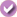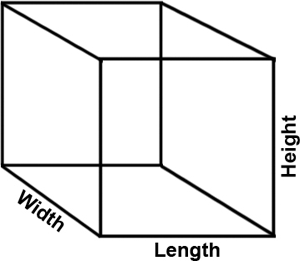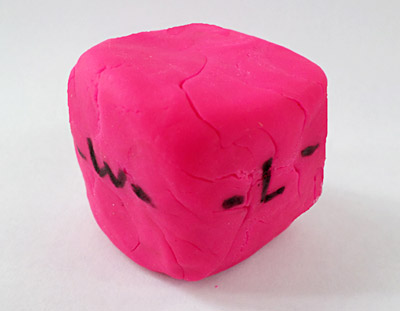# Play-Doh Math

 Areas of Science Pure Mathematics Difficulty Time Required Very Short (≤ 1 day) Prerequisites None Material Availability Readily available Cost Very Low (under \$20) Safety No issues

## Abstract

Have you ever had fun making different figures or colorful creations using some Play-Doh? You can squish and stretch a single piece of Play-Doh® to make all sorts of shapes. How does changing the shape of a piece of Play-Doh affect its volume? In this science project, you will find out by testing how changing a piece of dough's shape affects its dimensions (length, width, and height), and how these changes are related to the dough's volume.

## Objective

Test how changing an object's dimensions (length, width, and height) affects its volume.

## Share your story with Science Buddies!Yes, I Did This Project! Please log in (or create a free account) to let us know how things went.

## Credits

Sara Agee, Ph.D., and Teisha Rowland, Ph.D., Science Buddies

General citation information is provided here. Be sure to check the formatting, including capitalization, for the method you are using and update your citation, as needed.

### MLA Style

Science Buddies Staff. "Play-Doh Math." Science Buddies, 12 Jan. 2020, https://www.sciencebuddies.org/science-fair-projects/project-ideas/Math_p019/pure-mathematics/play-doh-math?from=Blog. Accessed 19 Feb. 2020.

### APA Style

Last edit date: 2020-01-12

## Introduction

Geometry is the study of how to use math to describe and investigate different points, lines, and shapes. A very basic three-dimensional shape is the rectangular prism. A rectangular prism is a shape like a box or a book. It has six different sides, and if all six sides are the same length, then it is called a cube. A cube is the same shape as a die (i.e., one of a group of dice, like the ones shown in Figure 1, below), where each side is a perfect square. Cubes and rectangular prisms can be measured with the same geometrical, mathematical formulas.Figure 1. This picture shows several green dice. Each die is a cube, which is a type of rectangular prism.

A mathematical formula is the way a shape is described in geometry. A mathematical formula is simply a way to calculate different properties of a shape, such as its area and volume. Volume is a unique property of three-dimensional shapes because three-dimensional shapes take up space in three different directions: length, width, and height. These dimensions are labeled on a rectangular prism shown in Figure 2, below.Figure 2. This shows a drawing of a rectangular prism with its three dimensions labeled (width, length, and height).

In this mathematics science project, you will use Play-Doh to make a model of a rectangular prism. You will measure the three dimensions (length, width, and height) and use a formula to calculate the volume. You will change one dimension (height), by squishing the Play-Doh, and see what effect this has on the other two dimensions and on the object's volume.

## Terms and Concepts

• Three-dimensional (3-D)
• Rectangular prism
• Cube
• Mathematical formula
• Volume
• Length
• Width
• Height

### Questions

• How is a cube a type of rectangular prism?
• What are the dimensions of a rectangular prism?
• How is a three-dimensional object's width, length, and height related to its volume?

## Bibliography

This site is a really good site for kids to review math skills, solve puzzles and read stories about math. They also have a section on geometry:
You can use this resource to create a graph on a computer:

## News Feed on This Topic, ,
Note: A computerized matching algorithm suggests the above articles. It's not as smart as you are, and it may occasionally give humorous, ridiculous, or even annoying results! Learn more about the News Feed

## Materials and Equipment

• Play-Doh (one approximately fist-sized piece)
• Metric ruler
• A flat surface, such as a hard binder or book. Make sure you have permission to possibly get Play-Doh on the flat surface you use.
• Sheets of graph paper (at least 5 sheets) with lines that are 1 mm or 2 mm apart; available from office or school supply stores or you can print free graph paper online with lines that are 2 mm apart.
• A pen or pencil
• A permanent marker
• Lab notebook
.Play-Doh Math

#### https://www.sciencebuddies.org/science-fair-projects/project-ideas/Math_p019/pure-mathematics/play-doh-math

PDF date: 2020-02-20

## Experimental Procedure

1. Get out store-bought Play-Doh or make some using this simple homemade play dough recipe.
2. Take a chunk of dough about as large as your fist.
3. Make your dough into a cube shape, approximately square on all sides. You can use the ruler to make sure all sides are the same.
4. Using the permanent marker, label each of the three dimensions of the cube with a "W" (for "width"), "L" (for length), or a "H" (for height). Look back at Figure 2 in the Background section, or take a look at Figure 3, below, if you are not sure how to label these dimensions. On either side of each letter, put a dash to show the direction of the dimension on that side of the cube.Figure 3. This Play-Doh cube has been labeled with a "– W –" to mark its width and a "– L –" to mark its length. It has a "H" (with vertical dashes above and below the letter) on one of its back sides to mark its height.
1. Place your dough on the graph paper and measure all three dimensions (length, width, and height). Do this by tracing along the cube's edge on the graph paper. Be sure to label each tracing as "length," "width," or "height." Write down the words "Trial #1" on the top of the sheet of graph paper.
2. After you have measured the 3 dimensions, you are ready to change the shape of your dough by doing the following:
1. Put the dough cube on a flat surface with the side marked for the height pointing up and down (the dashes should be vertical).
2. Put a flat surface, such as a book or a hard binder, on top of the dough cube.
3. Slowly press down on the dough. While you do this, try to keep the corners square (i.e., straight) as you go by patting in from the sides with your hands. Stop pressing down when it looks like the cube has changed shape a little (you do not want to completely squish the cube into a pancake yet!).
4. The letters and marks you made on the dough should stay there even though they may have been squished or stretched as you pressed down on the dough. If they begin to disappear, mark over them again with the permanent marker.
3. Repeat step 5 with a new piece of graph paper. Write down the words "Trial #2" on the top of the sheet of graph paper.
4. Repeat steps 5–7, pressing down on the dough a bit more each time between measurements. Remember to keep the corners square as the cube becomes flat. Continue to measure each dimension after pressing down on the dough and write the data on a new piece of graph paper labeled on the top with "Trial #___" until you have 5 different measurements (a total of 5 trials).
5. In your lab notebook, make a data table like Table 1, below. In your data table, write down how long the tracings are that you made on each sheet of graph paper (in centimeters [cm]).
1. You can use the lines on the graph paper to figure out how long the tracings are (note that 10 millimeters [mm] equals 1 cm), or you can use a metric ruler to measure the tracings.
Trial
Number
Length
(cm)
Width
(cm)
Height
(cm)
Volume (cm³)
V = L × W × H
1
2
3
4
5
Table 1. In your lab notebook, make a data table like this one. Write down how long the length, width, and height of the dough is for each trial you did (as you pressed down on the dough and squished it).
1. For each trial, multiply the length × width × height to calculate the volume of the shape. In your lab notebook, write down the volumes you calculate for each trial (in centimeters cubed, or cm³).
2. From the data table, make two graphs of your results. Make one graph showing the length, width, and height measurements you took, and make a second graph showing the volume measurements.
1. The best type of graph for this experiment is a bar graph.
1. On one graph, put the type of measurements you took (length, width, or height) on the horizontal axis (the x-axis), and put how long the measurements were (in cm) on the vertical axis (the y-axis). Put all of your trials on the same graph, but color-code the bars that belong to the same trial.
2. On a second graph, put the trial numbers for each bar on the x-axis, and your calculations for the volume (in cm3) on the y-axis. Make each bar a different color, and match the colors for the trials to the first graph you made.
2. You can make your graph by hand or you can try using the Create a Graph web site for kids from the National Center for Education Statistics.
3. Analyze your results. What happens as one dimension (the one you flattened) decreases? Do the other two dimensions increase or decrease? Does changing the dimensions of the dough affect its volume, or does the volume stay the same?
.

## If you like this project, you might enjoy exploring these related careers:

### Mathematician

Mathematicians are part of an ancient tradition of searching for patterns, conjecturing, and figuring out truths based on rigorous deduction. Some mathematicians focus on purely theoretical problems, with no obvious or immediate applications, except to advance our understanding of mathematics, while others focus on applied mathematics, where they try to solve problems in economics, business, science, physics, or engineering. Read more

### Math Teacher

Math teachers love mathematics and understand it well, but much more than that, they enjoy sharing their enthusiasm for the language of numbers with students. They use a variety of tools and techniques to help students grasp abstract concepts and show them that math describes the world around them. By helping students conquer fears and anxieties about math, teachers can open up many science and technology career possibilities for students. Teachers make a difference that lasts a lifetime! Read more

## Variations

• In this experiment you changed the length, width, and height of a cube of dough, but you did not change the amount of dough you used. What would happen if the amount of dough did change? Would the volume also change? Try the experiment adding or taking away some dough, forming the dough into a cube and measuring the dimensions of the shape.
• In this science project, you collected data from 5 different trials. For a more advanced science project, you could graph the relationship between the side you are flattening and the other two sides. Make an X,Y dot plot of the height vs. width, height vs. length, or height vs. width + length. Then you can draw a line of best fit. What is the equation for that line? What does this tell you about the relationship between the measurements of a three dimensional rectangular shape under constant volume? You can make your graphs by hand or you can try using the Create a Graph web site for kids from the National Center for Education Statistics.
• In this science project we used a three dimensional rectangular shape. For a more advanced project, try this experiment with other more complex shapes, like a pyramid, cylinder, or sphere. You can download a program like Geometry by Travis East to help you.

## Share your story with Science Buddies!Yes, I Did This Project! Please log in (or create a free account) to let us know how things went.

The Ask an Expert Forum is intended to be a place where students can go to find answers to science questions that they have been unable to find using other resources. If you have specific questions about your science fair project or science fair, our team of volunteer scientists can help. Our Experts won't do the work for you, but they will make suggestions, offer guidance, and help you troubleshoot.